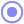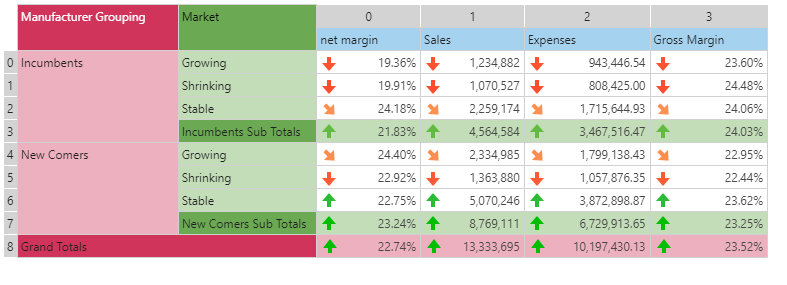#Case (PQL)

This logical function evaluates a list of logical expressions, returning the a result that matches the first true expression in the list.

• Returned Output: Variant
• Library: PQL \ Common \ Logical
• Version: 2018.00.000
• Compatibility:
• Can be combined with and other PQL function throughout the application.
• It CANNOT be used with MDX or VBA functions. But it can be used on MDX-based content in other parts of the application.

#### Syntax

Case(, , <Default> )

* Click on the function's arguments above for more details on the input values.

• The case function has 3 parts: criteria, results / outcomes and default:
• The criteria set is a comma delimited collection of logical statements
• The results (or outcomes) set is a comma delimited collection of alphanumeric values that match each item in the criteria set
• The default value is a single alphanumeric item - used if no criteria is met.
##### Different Function types
• This function is like an inline switch statement found in languages like VB, C#.
• Also see the If function.

#### Examples

This example uses 3 logical statements as the case criteria: 1=9, 2=2, 3=4. The function checks each statement and returns the corresponding outcome from the results list: 1,2,3. The first true statement is 2=2, so the function returns 2, which is the second outcome.

case( criteria(1=9,2=2,3=4), criteriaResults(1,2,3), 6 )

This example uses 3 logical statements as the case criteria: 1<9, 2=2, 3=4. The first true statement is 1<9, so the function returns "a", which is the first outcome.

case( criteria(1<9,2=2,3=4), criteriaResults("a","b",3), 50 )

This example uses 3 logical statements as the case criteria: 1>9, 2!=2, 3=4. None of the statements are true, so the function returns 50 - the default value.

case( criteria(1>9,2!=2,3=4), criteriaResults("a","b",3), 50 )

This example uses the dynamic grid below.

case( criteria( data(0,0)<average(dataSetColumn(0)), data(8,1)>500, data(8,0)=data(8,3) ), criteriaResults("good","bad","ugly"), "unknown" )

The first criteria checks if data point (0,0) is less than the average of the values in column 0. So it compares 19.36% to the average of net margin across all cells. The second criteria checks if the grand total of sales, data point (8,1), or 13,333,695 is greater than 500. And the last criteria checks if total net margin (8,0) is equal to total gross margin (8,3).

Since the first statement turns out to be true, the function resolves to "good".